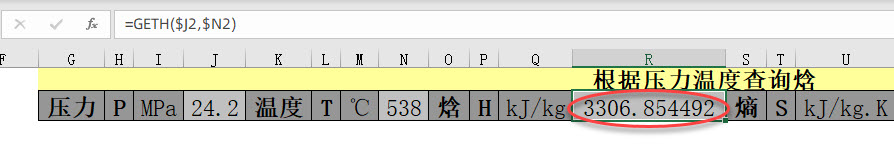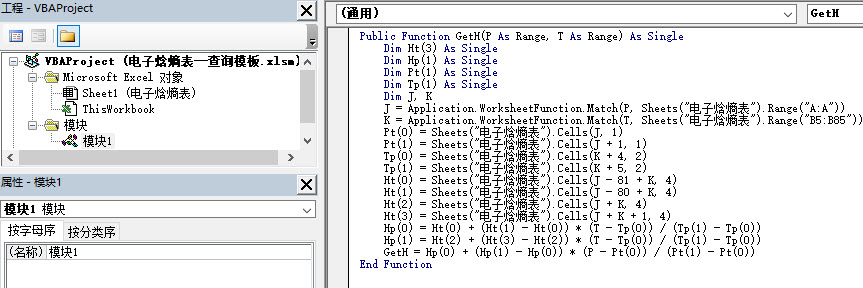﻿ excel、wps中出现的非内置不可识别函数是怎么回事？ - EXCEL VBA - ExcelOffice【微信公众号：水星Excel】

# excel、wps中出现的非内置不可识别函数是怎么回事？GETH这个函数在Excel中是找不到的。

```Public Function GetH(P As Range, T As Range) As Single
Dim Ht(3) As Single
Dim Hp(1) As Single
Dim Pt(1) As Single
Dim Tp(1) As Single
Dim J, K
J = Application.WorksheetFunction.Match(P, Sheets("电子焓熵表").Range("A:A"))
K = Application.WorksheetFunction.Match(T, Sheets("电子焓熵表").Range("B5:B85"))
Pt(0) = Sheets("电子焓熵表").Cells(J, 1)
Pt(1) = Sheets("电子焓熵表").Cells(J + 1, 1)
Tp(0) = Sheets("电子焓熵表").Cells(K + 4, 2)
Tp(1) = Sheets("电子焓熵表").Cells(K + 5, 2)
Ht(0) = Sheets("电子焓熵表").Cells(J - 81 + K, 4)
Ht(1) = Sheets("电子焓熵表").Cells(J - 80 + K, 4)
Ht(2) = Sheets("电子焓熵表").Cells(J + K, 4)
Ht(3) = Sheets("电子焓熵表").Cells(J + K + 1, 4)
Hp(0) = Ht(0) + (Ht(1) - Ht(0)) * (T - Tp(0)) / (Tp(1) - Tp(0))
Hp(1) = Ht(2) + (Ht(3) - Ht(2)) * (T - Tp(0)) / (Tp(1) - Tp(0))
GetH = Hp(0) + (Hp(1) - Hp(0)) * (P - Pt(0)) / (Pt(1) - Pt(0))
End Function```